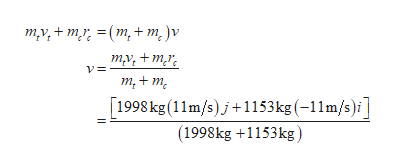# A car with mass mc = 1153 kg is traveling west through an intersection at a magnitude of velocity of vc = 11 m/s when a truck of mass mt = 1998 kg traveling south at vt = 11 m/s fails to yield and collides with the car. The vehicles become stuck together and slide on the asphalt, which has a coefficient of friction of μk = 0.5. (a)  Write an expression for the velocity of the system after the collision, in terms of the variables given in the problem statement and the unit vectors i and j. (b)  How far, in meters, will the vehicles slide after the collision?

Question
2035 views

A car with mass mc = 1153 kg is traveling west through an intersection at a magnitude of velocity of vc = 11 m/s when a truck of mass mt = 1998 kg traveling south at vt = 11 m/s fails to yield and collides with the car. The vehicles become stuck together and slide on the asphalt, which has a coefficient of friction of μk = 0.5.

(a)  Write an expression for the velocity of the system after the collision, in terms of the variables given in the problem statement and the unit vectors i and j.

(b)  How far, in meters, will the vehicles slide after the collision?

check_circle

Step 1

The mass of the car is 1153kg, moving with a speed of 11m/s, and the mas of truck is 1998kg, moving with a speed of 11m/s.

Step 2

(a)The collision is inelastic. Hence the final velocity of car and truck after collision will be v.

Write the momentum conservation equation.help_outlineImage Transcriptioncloseту,+ my, 3D(m, + m,)v тy, + mr. т,+ m, [1998 kg(11m/s)j+1153kg (-11m/s)i] (1998kg +1153kg) fullscreen
Step 3

(b) Write the expression for kinet...

### Want to see the full answer?

See Solution

#### Want to see this answer and more?

Solutions are written by subject experts who are available 24/7. Questions are typically answered within 1 hour.*

See Solution
*Response times may vary by subject and question.
Tagged in
SciencePhysics

### Work,Power and Energy